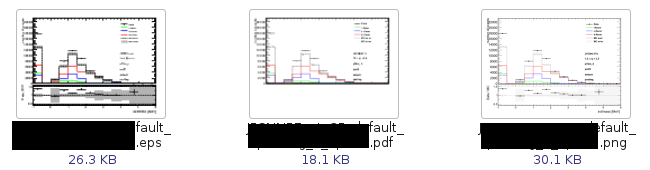# TCanvas->SaveAs("*.pdf")

Hello everyone!

I am using a TCanvas, plotting two TPads on it (top and bottom) and plotting TH1F on those two TPads.

In the end I save the canvas as *.png, *.eps and *.pdf. The *.png and *.eps images look fine, but the *.pdf is cutting away about 1/3 of the plot (see screenshot). Is there any known bug/issue/common mistake known for this behaviour!? Since the *.png and *.eps images look fine, my script can’t be (too) wrong!?

Minimal (but non-running, since histograms not declared here) code example:

``````TCanvas c("canvas", "canvas", 1024, 768);

c.cd();

h1_tpl.Draw("");
h1_mc_l.Draw("SAME HIST");
h1_mc_c.Draw("SAME HIST");
h1_mc_b.Draw("SAME HIST");
h1_mc_sum_err.Draw("SAME E2");
h1_mc_sum.Draw("SAME HIST");
h1_data.Draw("SAME");

c.cd();

h1_ratio_tpl.Draw();
h1_ratio_err.Draw("SAME E2");
h1_ratio.Draw("SAME");

c.cd();

legend.Draw();

l.DrawLatex(0.70, 0.60, latex1);
l.DrawLatex(0.70, 0.55, latex2);
l.DrawLatex(0.70, 0.50, latex3);
l.DrawLatex(0.70, 0.45, latex4);
l.DrawLatex(0.70, 0.40, latex5);
l.DrawLatex(0.70, 0.35, latex6);

c.Update();

c.SaveAs("name.png");
c.SaveAs("name.eps");
c.SaveAs("name.pdf");

c.Close();``````I would need a running one to investigate.

As always it is hard to provide a minimal working example reproducing exactly the same conditions, since this part of code is included into a larger code package. Therefore creating a minimal example with filling the histograms with TH1F::FillRandom(…) produces proper plots also for *.pdf output format, contrary to my code segment…

So the question is, what is different for calling the TCanvas::SaveAs(…) method with .eps/.png or *.pdf as output format, since the first two work properly with my code, which seems to contain a bug which only appears when saving the canvas as *.pdf …

Cheers
HeXor

PS: One can hardly see this from the screenshot, but the bottom Pad is not just “missing” or “not plotted”, it is cut away as if the bottom margin of the bottom Pad had a negative value…

They are 3 different drivers.
Which ROOT version are you using ?

I am using root_v5.30.04_64

Thanks, that’s quite old. We are now at 5.34.
Also instead of sending me a running macro you can send me the root file corresponding to

C->SaveAs(“c.root”);

Here is the output you requested
c.root (17.1 KB)

It looks lik the faulty code is in you macro is:

h1_mc_sum_default_posttag_l_pt020to030__1->GetXaxis()->SetLabelSize(1e-07);

Well since I am adding a ratio plot, and since I want to put this ratio plot right below the other plot, I would like to hide the x-axis labels of the top plot, which else would half-appear behind the ratio plot. This line of code was the easiest and quickest way to provide this.

Do you have any suggestions how this can be done in cleaner way to avoid the *.pdf output issues?

You should set the size to 0 instead of 1e-07.
Pdf (and PS) have some precision issues.

As easy as it sounds,
thank you very much!